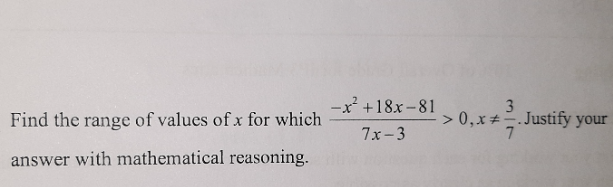# Questionis there no solution ? is x > 3/7 accepted ?

Sorry my bad.  I have only considered 1 scenario in the previous solution so I have unaccepted my own solution.

For  [-x2 + 18x – 81 ] /  [7x – 3] > 0, we have to consider 2 scenarios.

N = numerator, D = denominator

Case 1:  N > 0 and D > 0

This is the case where there is no solution.

-x2 + 18x – 81 > 0

=> – (x2 – 18x + 81)> 0

=> -(x-9)2 >  0

However, for all values of x (+ve or -ve or 0) except x =9, the expression is always a negative number and can never be > 0, hence no solution for x.   So even if  D which is 7x-3 > 0 where x > 3/7, there is no solution for x in this case.

Case 2:  N < 0 and D < 0

This is the case with a solution.

-x2 + 18x – 81 <  0

=> – (x2 – 18x + 81) < 0

=> -(x-9)2  <  0

For all values of x except x = 9 in this case, the expression is always a negative number and hence it is true that it is always < 0.  So let’s check the D to narrow down the range of x.

7x-3 < 0

=> 7x < 3

=> x < 3/7

Hence the solution is x < 3/7

Thank you @mr.tan

```Numerator = -x2 + 18x - 81 = -(x2 - 18x + 81) = -(x - 9)2 <= 0
for all values of x, with equality if and only if x = 9.

Case 1: x = 9
Numerator is 0 so 0 > 0 cannot be satisfied.

Case 2: x != 9
Numerator is always < 0. So the expression is true if and
only if the denominator 7x - 3 < 0.

Hence x < 3/7 is the range of values of x for which the
expression is always true. (Note: x=9 is not in the range.)

See https://www.geogebra.org/graphing/fq35qqa2 for a graphical explanation.

- The reason why the question stated x != 3/7 is to prevent
7x - 3 = 0. The function is not well-defined at x = 3/7 if
we have division by 0.

- Your guess x > 3/7 is a good one (shows you have good intuition)
but you need to take into account the signs of the expressions.

- numerator/denominator > 0 means numerator and denominator are
the same signs, i.e. both positive or both negative (e.g. 1/2, -1/-2).

- numerator/denominator < 0 means they are opposite signs (e.g. 1/-2, -1/2).

This question can be made more interesting by asking for range of x where
the expression is always < 0. (Ans: 3/7 < x < 9 OR x > 9)```

0 Replies 1 Like